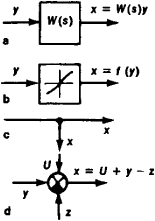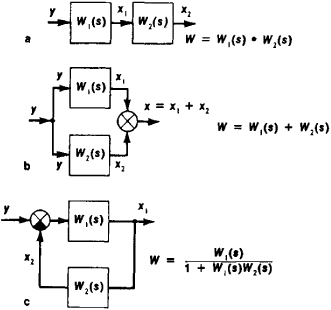Block Diagram, Automatic-Control

The following article is from The Great Soviet Encyclopedia (1979). It might be outdated or ideologically biased.

Block Diagram, Automatic-Control

a graphic representation of an automatic control system in the form of a set of components into which the system may be divided according to certain features and a set of connections between the components; it also shows the direction of the transfer of signals. Block diagrams of control systems are generally constructed according to structural, functional, or algorithmic design. Algorithmic block diagrams are the type most often used for automatic control systems; they give a complete representation of the dynamic properties of the system. An algorithmic block diagram of an automatic control system can be constructed according to the differential equations describing the system; conversely, given such a block diagram, the differential equations describing the system can be formulated.

A block diagram depicts an automatic control system’s components, connections, and branch points (the points where connections divide). The components are shown with their transfer functions or with the operators of the nonlinear transformations that the components carry out. The most important components with elementary algorithms include dynamic, forming, arithmetic, and logic components. Dynamic components alter an input signal over time; they include integrating, differentiating, and time-delay components. Forming components alter the scale and waveform of the input signal; they include proportional, modulating, and impulse components. Arithmetic units include additive and multiplicative types. Logic components carry out logic operations on input values. Linear dynamic and scalar components as well as function generators are represented in block diagrams by squares. Summators are represented by a circle divided into sectors; sectors for which subtrahends are given are often blackened. Branch points are represented by a heavy dot at the intersection of the corresponding connections (Figure 1).

The transfer function of an individual component or of an entire system is the ratio of the representation of the input value to that of the output value under conditions of zero initial-energy storage (seeLAPLACE TRANSFORM). The transfer function fully describes the dynamic properties of the system or of the system’s components. It is usually represented by W(s), W(p), or simply W (s or p represents the argument of a Laplace transform).Figure 1. Elements of block diagrams: (a) linear component, (b) function generator, (c) branch point, (d) summator. W(s) is a transfer function, y, U, and z are input signals, and x is an output signal.

Any linear segment of an automatic control system with lumped parameters may be broken down into elementary units that cannot be further resolved. There are four types of such units: integrating, differentiating, scalar, and additive. Real automatic control systems, in which the power of the numerator of the transfer function is not greater than the power of the denominator, may usually be represented by units of only three types (excluding differentiating units). The resolution into elementary units is convenient in modeling automatic control systems on analogue computers. In other methods of study, the linear segment is usually broken up into more complex units: first-order units (nonideal differentiating, integrating-differentiating, and aperiodic units) and second-order units (nonideal integrating, cycling, and time-delay units). The order of a linear unit of a block diagram is determined by the order of the dynamics of the differential equation describing the unit.

Since the transfer function of a system adequately describes the system’s dynamic properties, a block diagram may replace another, equivalent diagram with the single necessary and sufficient condition that the two transfer functions be equal. The transformation of ordinary linear block diagrams is governed by the rules of transformation of the simplest types of connections: series, parallel, and feedback (Figure 2). Block diagrams as a whole and units of any order greater than second may be replaced by several block diagrams of less than second order, which significantly simplifies the analysis and synthesis of an automatic control system.Figure 2. Simplest connections of linear units: (a) series, (b) parallel, (c) feedback. W,(s) is the transfer function of the ith unit, W is the transfer function of the system, y is an input signal, and x is an output signal.

In addition to block diagrams, oriented graphs are also used in the structural study of automatic control systems (seeGRAPHS, THEORY OF). The system’s parameters are placed accordingly at the vertices of the graphs, and transfer functions or operators of functional transforms are placed along the line segments linking the vertices.

REFERENCES

Petrov, B. N. “O postroenii i preobrazovanii strukturnykh skhem.” Izv. AN SSSR: Otdelenie tekhnicheskikh nauk, 1945, no. 12.
Solodov, A. V. Lineinye sistemy avtomaticheskogo upravleniia speremennymiparametrami. Moscow, 1962.
Shatalov, A. S. Strukturnye melody v teorii upravleniia i elektroavtomatike. Moscow-Leningrad, 1962.
Voronov, A. A. Osnovy teorii avtomaticheskogo upravleniia, parts 1–3. Moscow, 1965–70.
Tekhnicheskaia kibernetika: Teoriia avtomaticheskogo regulirovaniia, book 1. Edited by V. V. Solodovnikov. Moscow, 1967.

A. A. VORONOV